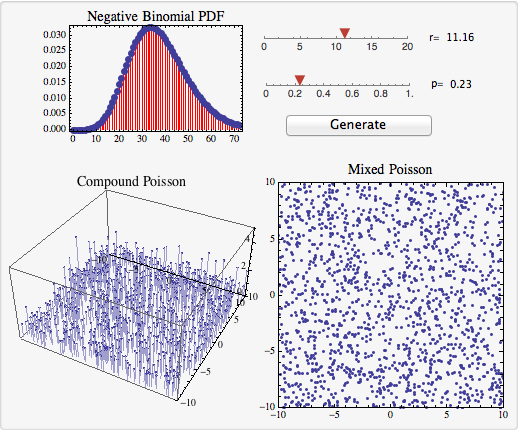# Limitations of the negative binomial distribution in spatial models

Galaxies, trees, and influenza cases have something in common: they tend to occur in clusters. The issue of how to model clustered spatial patterns is thus of interest to a variety of scientific disciplines. For many people, the mention of spatial clumping brings to mind the negative binomial distribution. This is appropriate, because the negative binomial distribution is overdispersed, meaning its variance is greater than its mean (in contrast to the Poisson distribution, which has equal variance and mean). Unfortunately, there are some difficulties in using the negative binomial distribution in spatial modeling, which we attempt to explain in this post.

Suppose that you want to create a model that generates spatial locations of trees. You want the model to be stochastic, so that each time you generate a forest, you end up with a different configuration of trees. The relevant tool is a spatial point process; mathematical details are described in books such as An Introduction to the Theory of Point Processes by Daley and Veres-Jones. Each forest that you generate is called a realization of the process.

Further suppose you mark out a particular region, and generate many different forests, each time counting how many trees fall within the region. If your model is realistic, then, for some of the forests, the selected region will be empty (you will count zero trees) while for other forests, a cluster of trees will fall in the region (and you will count a large number). A good model will produce a distribution of counts that is overdispersed.

So, should you use a negative binomial distribution for your model? No. The negative binomial distribution describes the distribution of counts of trees, but it does not tell you WHERE TO PUT THEM. The distribution is not itself a spatial point process. There are spatial point processes that are compatible with the negative binomial distribution; that is, there are models that tell you where to put the trees, and have a negative binomial distribution for the counts of trees in any selected region.

There are two simple examples:

1. A compound Poisson process. The locations of tree clusters are generated by a Poisson process. The number of trees per cluster is generated by a logarithmic distribution.

2. A mixed Poisson process. A parameter drawn from a gamma distribution is used as the intensity for a Poisson process that generates the tree locations.

The Wolfram CDF module below (download CDF here)1 illustrates these two processes. The number of trees in a unit area follows a negative binomial with parameters r and p; you can manipulate these parameters with the slider bars. Hitting the `Generate` button produces spatial patterns for trees according to the two processes.There are two things to notice. The plot for the compound process has an extra dimension: the height of the points indicates the number of trees that are located at that point. This is a problem, because it’s not very realistic to have multiple trees in the EXACT SAME LOCATION.

The mixed Poisson process has a different problem. The forests it generates are NOT CLUMPY. Even though the tree counts in a region follow a negative binomial distribution over a large number of realizations, the spatial distribution within a single realization is Poisson.

Mathematically, the compound process described above is not “orderly” (more than one tree can occupy the same location), and the mixed process is not “ergodic” (averages of a single realization over a large spatial scale are not the same as averages of a small region over many realizations). To model clumpy forests, we want a spatial point process that is orderly and ergodic. We also want the process to be stationary (the counts should be translation invariant, so that the counts are independent of location).

I have bad news for you: there are no stationary, orderly, ergodic spatial point processes that have negative binomial count distributions. This issue was first examined in a great paper by Peter Diggle. Something has got to give in your model, so what should you sacrifice? The logical choice is the negative binomial property. Try a model like a Neyman-Scott process that is clumpy, stationary, orderly, and ergodic. Problem solved!

1. To use the module, you will need the free CDF Player.↩︎